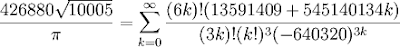# Hypergeometric series with SymPy

July 7, 2008

SymPy 0.6.0 is out, go get it! (It will be required for running the following code.)

Here is a nice example of what SymPy can be used for (I got the idea to play around with it today): automated generation of code for efficient numerical summation of hypergeometric series.

A rational hypergeometric series is a series (generally infinite) where the quotient between successive terms, R(n) = T(n+1)/T(n), is a rational function of n with integer (or equivalently rational) coefficients. The general term of such a series is a product or quotient of polynomials of n, integers raised to the power of An+B, factorials (An+B)!, binomial coefficients C(An+B, Cn+D), etc. The Chudnovsky series for π, mentioned previously on this blog, is a beautiful example:Although this series converges quickly (adding 14 digits per term), it is not efficient to sum it term by term as written. It is slow to do so because the factorials quickly grow huge; the series converges only because the denominator factorials grow even quicker than the numerator factorials. A much better approach is to take advantage of the fact that each (n+1)’th term can be computed from the n‘th by simply evaluating R(n).

Given the expression for the general term T(n), finding R(n) in simplified form is a straightforward but very tedious exercise. This is where SymPy comes in. To demonstrate, let’s pick a slightly simpler series than the Chudnovsky series:The SymPy function hypersimp calculates R given T (this function, by the way, was implemented by Mateusz Paprocki who did a GSoC project for SymPy last year):

>>> from sympy import hypersimp, var, factorial>>> var('n')n>>> pprint(hypersimp(factorial(n)**2 / factorial(2*n), n)) 1 + n-------2 + 4*n

So to compute the next term during the summation of this series, we just need to multiply the preceding term by (n+1) and divide it by (4n+2). This is very easy to do using fixed-point math with big integers.

Now, it is not difficult to write some code to automate this process and perform the summation. Here is a first attempt:

from sympy import hypersimp, lambdifyfrom sympy.mpmath.lib import MP_BASE, from_man_expfrom sympy.mpmath import mpf, mpdef hypsum(expr, n, start=0):    """    Sum a rapidly convergent infinite hypergeometric series with    given general term, e.g. e = hypsum(1/factorial(n), n). The    quotient between successive terms must be a quotient of integer    polynomials.    """    expr = expr.subs(n, n+start)    num, den = hypersimp(expr, n).as_numer_denom()    func1 = lambdify(n, num)    func2 = lambdify(n, den)    prec = mp.prec + 20    one = MP_BASE(1) << prec    term = expr.subs(n, 0)    term = (MP_BASE(term.p) << prec) // term.q    s = term    k = 1    while abs(term) > 5:        term *= MP_BASE(func1(k-1))        term //= MP_BASE(func2(k-1))        s += term        k += 1    return mpf(from_man_exp(s, -prec))

And now a couple of test cases. First some setup code:

from sympy import factorial, var, Rational, binomialfrom sympy.mpmath import sqrtvar('n')fac = factorialQ = Rationalmp.dps = 1000  # sum to 1000 digit accuracy

Some formulas for e (source):

print hypsum(1/fac(n), n)print 1/hypsum((1-2*n)/fac(2*n), n)print hypsum((2*n+1)/fac(2*n), n)print hypsum((4*n+3)/2**(2*n+1)/fac(2*n+1), n)**2

Ramanujan series for π (source):

print 9801/sqrt(8)/hypsum(fac(4*n)*(1103+26390*n)/fac(n)**4/396**(4*n), n)print 1/hypsum(binomial(2*n,n)**3 * (42*n+5)/2**(12*n+4), n)

Machin’s formula for π:

print 16*hypsum((-1)**n/(2*n+1)/5**(2*n+1), n) - \       4*hypsum((-1)**n/(2*n+1)/239**(2*n+1), n)

A series for √2 (Taylor series for √(1+x), accelerated with Euler transformation):

print hypsum(fac(2*n+1)/fac(n)**2/2**(3*n+1), n)

Catalan’s constant:

print 1./64*hypsum((-1)**(n-1)*2**(8*n)*(40*n**2-24*n+3)*fac(2*n)**3*\    fac(n)**2/n**3/(2*n-1)/fac(4*n)**2, n, start=1)

Some formulas for ζ(3) (source):

print hypsum(Q(5,2)*(-1)**(n-1)*fac(n)**2 / n**3 / fac(2*n), n, start=1)print hypsum(Q(1,4)*(-1)**(n-1)*(56*n**2-32*n+5) / \    (2*n-1)**2 * fac(n-1)**3 / fac(3*n), n, start=1)print hypsum((-1)**n * (205*n**2 + 250*n + 77)/64 * \    fac(n)**10 / fac(2*n+1)**5, n)P = 126392*n**5 + 412708*n**4 + 531578*n**3 + 336367*n**2 + 104000*n + 12463print hypsum((-1)**n * P / 24 * (fac(2*n+1)*fac(2*n)*fac(n))**3 / \    fac(3*n+2) / fac(4*n+3)**3, n)

All of these calculations finish in less than a second on my computer (with gmpy installed). The generated code for the Catalan’s constant series and the third series for ζ(3) are actually almost equivalent to the code used by mpmath for computing these constants. (If I had written hypsum earlier, I could have saved myself the trouble of implementing them by hand!)

This code was written very quickly can certainly be improved. For one thing, it should do some error detection (if the input is not actually hypergeometric, or if hypersimp fails). It would also be better to generate code for summing the series using binary splitting than using repeated division.

To perform binary splitting, one must know the number of terms in advance. Finding out how many terms must be included to obtain a accuracy of p digits can be done generally by numerically solving the equation T(n) = 10-p (for example with mpmath). If the series converges at a purely geometric rate (and this is often the case), the rate of convergence can also be computed symbolically. Returning to the Chudnovsky series, for example, we have

>>> from sympy import *>>> fac = factorial>>> var('n')n>>> P = fac(6*n)*(13591409+545140134*n)>>> Q = fac(3*n)*fac(n)**3*(-640320)**(3*n)>>> R = hypersimp(P/Q,n)>>> abs(1/limit(R, n, oo))151931373056000>>> log(_,10).evalf()14.1816474627255

So the series adds 14.18165 digits per term.

With some more work, this should be able to make it into SymPy. The goal should be that if you type in a sum, and ask for a high precision value, like this:

>>> S = Sum(2**n/factorial(n), (n, 0, oo))>>> S.evalf(1000)

then Sum.evalf should be able to automatically figure out that the sum is of the rational hypergeometric type and calculate it using the optimal method.